# Whittaker equation

(diff) ← Older revision | Latest revision (diff) | Newer revision → (diff)

A linear homogeneous ordinary differential equation of the second order: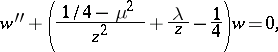(*)

where the variables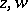and the parametersmay take arbitrary complex values. Equation (*) represents the reduced form of a degenerate hypergeometric equation and was first studied by E.T. Whittaker . Forthe Whittaker equation is equivalent to the Bessel equation. If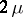is not an integer, a fundamental system of solutions of the Whittaker equation consists of the functionsand; here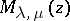is the Whittaker function (cf. Whittaker functions). For any value of the parameters the general solution of the Whittaker equation may be written in the form of a linear combination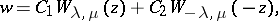where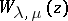is the Whittaker function.

How to Cite This Entry:
Whittaker equation. Encyclopedia of Mathematics. URL: http://encyclopediaofmath.org/index.php?title=Whittaker_equation&oldid=12547
This article was adapted from an original article by N.Kh. Rozov (originator), which appeared in Encyclopedia of Mathematics - ISBN 1402006098. See original article